# Gestep Function In Excel

• Last Updated : 09 May, 2021

GESTEP is a function in Microsoft Excel that is used to check whether the given number is greater than the given threshold value(given step value). It returns 1 i.e.  True , if the number is greater than the step value and, It returns 0 i.e. False , if the number is lesser than the step value.

Formula of GESTEP function:

`=GESTEP(number, [step])`

Here,

•  Number= given number that is to be tested.
• Step=defines the step size with which given number is compared. Step is completely optional. If step value is not given it is considered as 0

Example:

Lets suppose we have following numbers and steps, and we have to use the GESTEP function.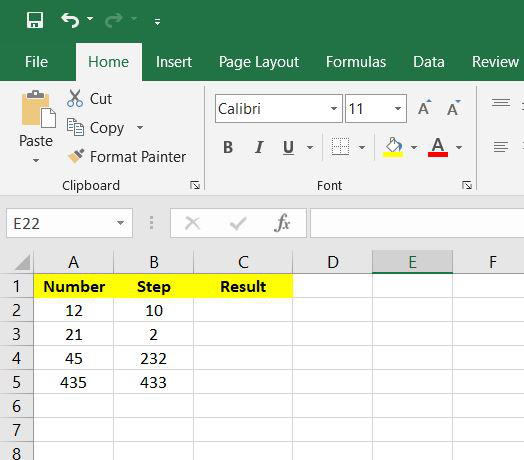Step 1: Now we will write =GESTEP(A2,B2), here A2 and B2 are the number and step values respectively. Now press enter.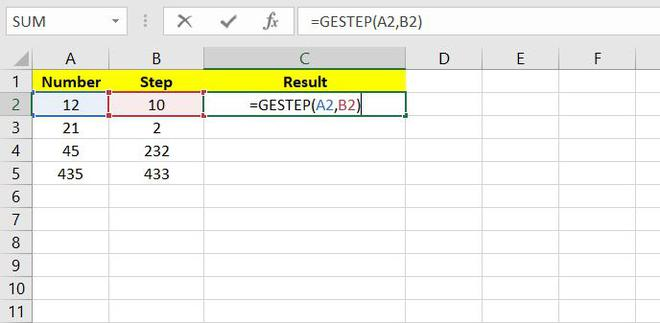Step 2: Now we can say that the Result value is 1 i.e. True . This is because the number value>Step value.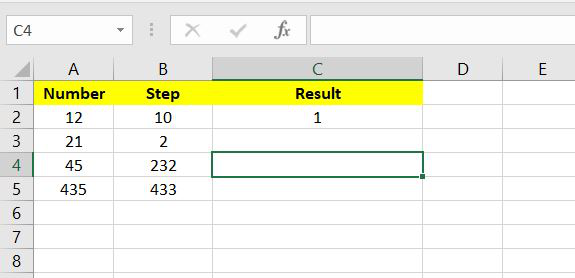Step 3: Now Drag and select from C2 to C5 and all the values for each row will be automatically calculated.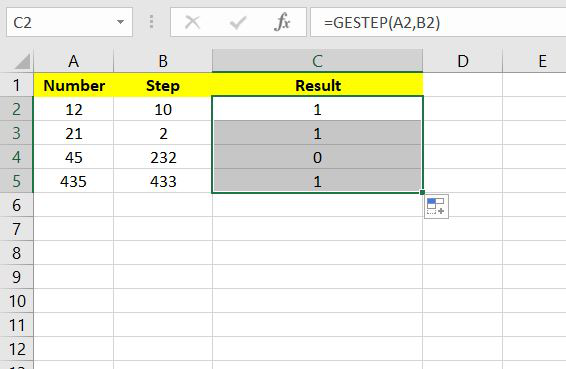Step 4: This is the final result of the given question.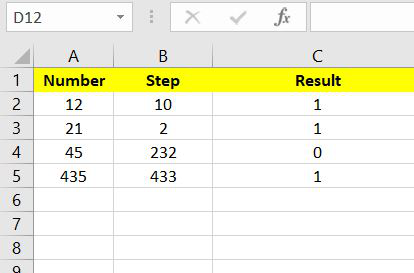### Common error:

#VALUE! =  This occurs if any of the values or both the values in the GESTEP function are non-numeric.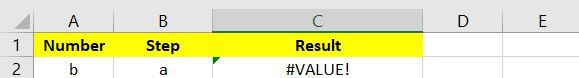My Personal Notes arrow_drop_up